#### Python Programming

This tutorial is ideal for:

• Those who do Python as a part of GCSE Computer Science course- OCR, AQA and Edexcel
• Those who do Python as a part of AS/A Level Computer Science course - OCR, AQA and Edexcel
• A complete novice who wants to learn the nitty-gritty of computer programming in Python
• Those who want to write data to a text file and read from a text file
• Those who want a springboard for OOP - Object Oriented Programming
• Those who want to learn Python quickly on this page - interactively on a real Python Console

Python is an insanely simple programming language to learn, mimic real-life situations and develop high level applications in an elegant manner; it is a language loved by Google too. If you have a passion for programming, this tutorial is a springboard for reaching the ultimate goal, even if you are a complete beginner. If you are a seasoned programmer from another background, this is ideal for a new start.

First of all, here is the link to download the latest Python for a Windows PC: once installed, choose Python IDLE - Integrated DeveLopment Environment or Integrated Development and Learning Environment - from the programmes list. then, follow the instructions in the following animation to write your first piece of code and make it work. Please remember the code you see here will not be part of it!

You will see Python Shell Window, which looks like the following: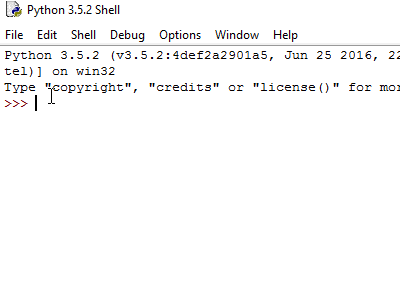This is also called, interactive mode, because you can write Python commands here, run them by pressing the Enter key and see them at work on the same screen. For instance, in the above animation, I showed how to add, multiply and find the absolute value of a number by using Python commands. Python commands are written next to the primary prompt - >>>. The result is immediately shown just below the primary prompt.

In addition, you can open a Python file, write the commands in it, save it as a .py file and then execute it too - the more professional approach. The file thus saved, can be opened up later to amend the code and re-run it, which is as follows:The first part of this tutorial is going to be in the interactive mode - the easier phase.

#### Programming in Interactive Mode

In interactive mode, Python shell can be turned into a versatile calculator, which is really fun! Here is how:

Let's, for instance, calculate the volume of a cylinder first:Here are some examples for you to practise with the interpreter in the interactive mode - in order to be familiar with Python basic operators: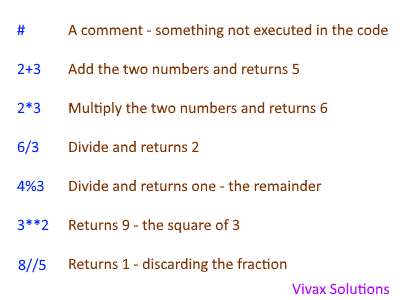#### It's time to practise interactively; here is the console!

You may experiment with the code samples on this page - or your own - on the console and see the results straightaway. If the screen gets clogged up, please typeclear and hit Enter on the keyboard. It'll clear up the screen.

#### Variables - the containers in memory!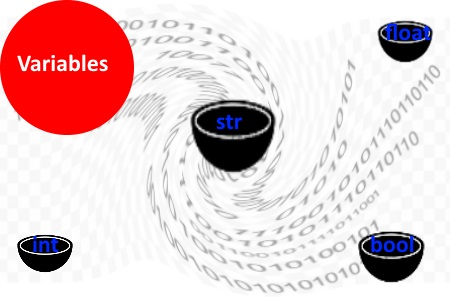Variables store the information or data in the memory and act as containers. The type of container determines what it can store. The three main types of variables are:

• str - e.g. "Hello!", for string variables
• float - e.g. 21.34, for floating point numbers
• int - e.g. 3, for integers

In addition, there are a few other types: bool - e.g. true/false, Boolean.

In real life, if a sieve is a container, it cannot hold water, but it can hold ice. Exactly in the same way, an integer type cannot hold a string or vice versa. In short, the variable type does matter, when it comes to storing data.

The mechanism of a variable in programming is shown below:As you can see, by knowing the exact data type, you can choose the right variable to store and manipulate it inside a programme.

The following animation shows how to determine the variable type by Python's built-in type()function:In sieve-water analogy, water cannot be held in a sieve, but ice can be held if water can be turned into ice. In the same way, Python allows us to convert data from one type to another easily and then deal with them. In the following example, an integer is converted into a string and then back into an integer: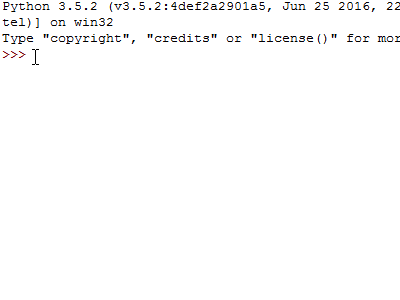Note: the words followed by # is treated as a comment by Python; so, that part is not executed.#### = means assignment, not equal in programming

The role of the equal sign, =, in programming is a confusing one for beginners. It is an assignment, as shown in the following code: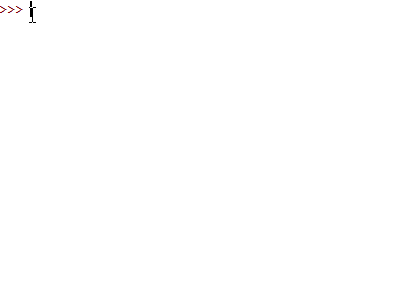In short, = does not behave as the equal sign of an equation, that could lead to a bizarre outcome: a=a+6 => 0=6!

#### Mathematical Operations Involving Assignment(=)

Here are a few simple mathematical operations that could be done with the assignment sign: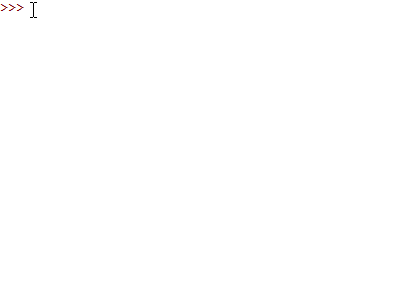The same can be performed with multiplication - * - and division - / - too.

#### String Concatenation

In Python, strings can be manipulated to produce the desired outcomes. It is known as string concatenation.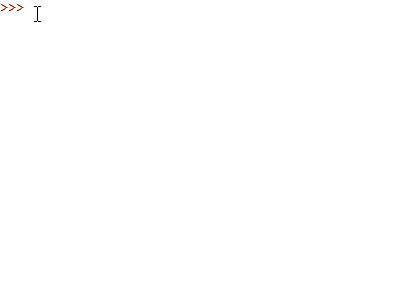In the above example, two words are assigned to the variables, a, and b. When the two variables are added, the string is concatenated to produce the combined phrase we want.#### String Manipulation

In Python, each character of a string has an index, as illustrated below:

E.g.
String: |B|e|n| |S|h|e|r|m|a|n|
Index: |0|1|2|3|4|5|6|7|8|9|10|
The index of B is 0, that of space is 3 and that of m is 8.

The following interactive programme shows how a string in manipulated by using the index positions of a string; you may run it by commenting out the line by line to learn the string manipulation.

#### Sub Routines

Sometimes, the programming code of the main programme can be very long, which in turn poses certain challenges: the difficulty in maintaining or updating; the repetitive nature of some parts of code. In order to address this issue, sub-routines come to our rescue. There are two kinds of sub-routines:

• ☛ Procedures
• ☛ Functions

#### Procedures

A procedure is something that the main programme can call many times to execute a certain task; it does not return a value.

E.g.

The following programme uses a procedure, printNumber() to print numbers from 1 - 10, when the main programme calls it.

import time

def printNumber(number): # procedure
print("This is the number: " + str(number))

count = 0 # main programme
while count<10:
count=count+1
printNumber(count)
time.sleep(1) # print with a time gap of 1 second.

The output is as follows:

This is the number: 1
This is the number: 2
This is the number: 3
This is the number: 4
This is the number: 5
This is the number: 6
This is the number: 7
This is the number: 8
This is the number: 9
This is the number: 10

If you want to practise interactively, please use the following; change the value of count in the while loop:

N.B. The procedure must precede the main programme; otherwise, it will throw an error. You may swap the positions around and try it!

#### Functions

Those of us who use calculators know the use of functions quite well text-muted: for instance, in order to find the square root of a number, we use the button with the sign, , to invoke the function that gives the square root of the number in question. The number that is entered into the function is called the parameter. The function is said to be returning the value of the number, when pressed. The simplified mechanism is as follows:
square root(number)
inner working hidden from the user
returning the square root as the output

The structure and the working of a function in Python is the same: we see the input and output, with the inner working being hidden from the user, unless you are a programmer. Here are some of the Python built-in functions at work: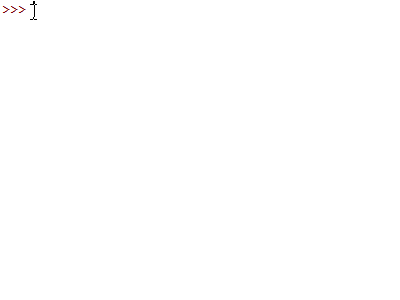A Python function to find the average of two numbers can be formed as follows:

In the above function, the def keyword defines the function and a and b are the parameters. The function returns the average of the two parameters, when entered into it, once it is being called; please note the indentation of the code, as it is really important in Python.

You may change the two parameters to see the function at work.

#### Loops

while loop: The following function shows how a while loop works;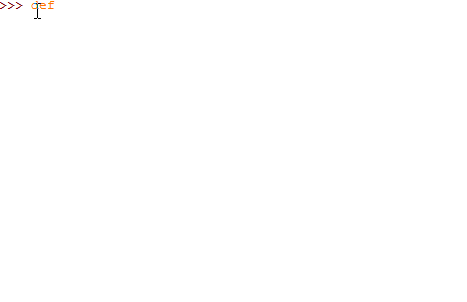The parameter, a, in the function accepts a number - the seed - and then print the number, increases it by 1 and stops working when the condition of the while is met.

for Loop: The following function shows the use of for loop:In the first function, range(4) works from 0 to 3. In the second function, range(2,8) works from 2 to 7.

if loop: if loop is illustrated in the following function: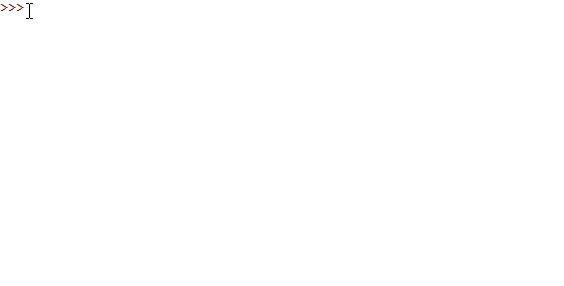The above function shows how if loop works; please note how python check the equality with two equal signs.Decision making is an important element in computer programming. This is how Bill Gates described its significance:

#### Factor Finder

With the following code, you can find all the factors of any given number.

def Factor_Finder(number):
chain=""
for i in range(1,number):
if number%i==0:
chain+=str(i)+" , "
chain+=str(number)
return chain
print(Factor_Finder(60))

The output is as follows:

1 , 2 , 3 , 4 , 5 , 6 , 10 , 12 , 15 , 20 , 30 , 60

#### Pyramid Maker

The following programme prints a triangle of stars, given the number of rows in a function.

n=int(input("How many rows of stars do you want? "))
for i in range(1,n+1):
print ((n-i)*' '+i*'* ')

It uses the simple Python programming trick as follows, in a print statement:
1*'*' = *
2*'*' = **
3*'*' = ***
Here is the output for 15 rows: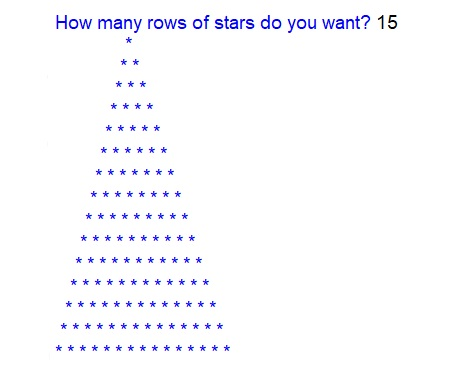Do you want to practise interactively? Here it is:

☕ Odd_Even_Checker

This is a fairly advanced function that checks whether the user input is odd or even with the aid of if loop.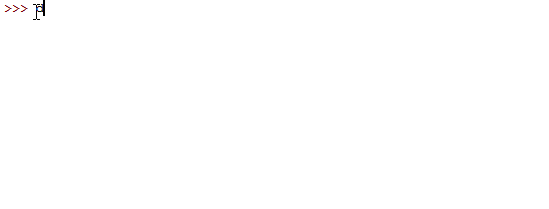Here is the full code for the above function:

def odd_even_checker():
if a%2 ==0:
print("It's an even number.")
else:
print("It's an odd number.")

Recommended BooksAlthough these two books are revision guides, they are reliable substitutes for existing text books. The contents are organized very well for students to follow in an orderly manner and then learn the concepts. The diagrams perfectly complement the rich contents. The books provide much needed assistance for the students who desperately need user-friendly text books.

☕ Graphics with Python turtle Library

In this case, we have to import a famous Python module called, turtle, which has been especially created for graphics. In order to use the relevant functions, we have to make a reference to the library with the keyword, import, in the code.

Create the function, drawing_square(), inside Python shell as follows:

def drawing_squares():
import turtle # importing the module
trtl = turtle.Turtle() #making a turtle object of Turtle class for drawing
screen=turtle.Screen() #making a canvas for drawing
screen.setup(400,300) #choosing the screen size
screen.bgcolor('black') #making canvas black
trtl.pencolor('red') #making colour of the pen red
trtl.pensize(5) #choosing the size of pen nib
trtl.speed(1) #choosing the speed of drawing
trtl.shape('turtle') #choosing the shape of pen nib
trtl.forward(100) #top line
trtl.right(90)
#turn right through 900 trtl.forward(100) # right vertical line
trtl.right(90)
trtl.forward(100) # bottom line
trtl.right(90)
trtl.forward(100) # left vertical line
# information printing
trtl.penup()
# pause drawing trtl.setpos(-120,100)
move the pointer to new position trtl.pendown() # restart drawing trtl.pencolor('green')
trtl.write('Square - Vivax Solutions', font=("Arial", 16, "bold"))
# the author informaton trtl.penup()
trtl.ht() # draw a horizontal line
drawing_squares()
The above code, if properly run, produces the following image:☕ Fibonacci Sequence Generator

The following animation shows how to create a function to generate the famous Fibonacci Sequence in Python. The parameter, f, entered by the user, determines the size of the series.The full code is as follows:

def Fibonacci(f):
a,b=0,1
while b<f:
print(b)
a,b=b,a+b

☕ Polygon Maker

The above programme draws polygons, starting from a triangle to a decagon, using Python Turtle.

The following animation shows how to make a simple quadratic solver in Python:

THe complete code of the programme is as follows:

d=b**2-4*a*c
if d>=0:
e=(-b+d**0.5)/(2*a)
f=(-b-d**0.5)/(2*a)
if d<0:
print ('No real solutions!')
elif: d==0:
print ('x = ' + str(e))
else:
print ('x = ' + str(e) + ' or '+ str(f))

The corresponding flow chart for the programme is as follows: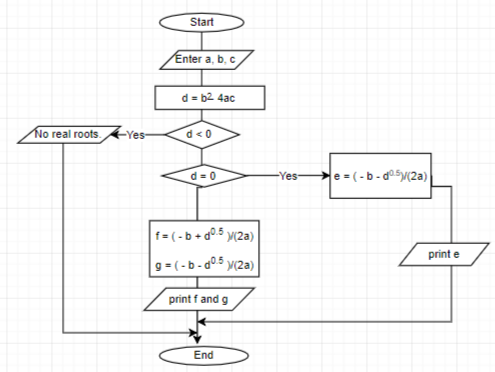Recommended BooksIf you want to practise what you learn here in depth, here is a book for you. It's well written and concise - a really good guide for novice programmers.This is a great book to expand your knowledge in Python: once you learn and master the basic concepts from this tutorial, this book will help you to broaden the understanding while practising more code challenges.

☕ Denary to Binary Converter

The following function takes the input of a denary number from a user and gives the output in binary form.

def denaryToBinary(): # function
nmbr=int(input("Enter the number: ")) # input in denary
number=nmbr
string=""
while number>0:
string=str(number%2)+string
if number>0:
number=number//2
return "The number, " + str(nmbr) + ", in binary form = " + string

print(denaryToBinary()) # calling the function

The output is as follows:

Enter the number: 107
The number, 107, in binary form = 1101011

The following function convert decimal numbers - denary - to hexadecimal form:

def denaryToHexa(): #function
nmbr=int(input("Enter the number: ")) #input in denary
number=nmbr
string=""
while number>0:
intmed=number%16
if intmed>=0 and intmed<=9:
string=str(intmed) + string
elif intmed==10:
string='A'+string
elif intmed==11:
string='B'+string
elif intmed==12:
string='C'+string
elif intmed==13:
string='D'+string
elif intmed==14:
string='E'+string
else
string='F'+string
if number>0:
number=number//16
return "The number, " + str(nmbr) + ", in hexadecimal form = " + string

print(denaryToHexa()) # calling the function

The output is as follows:

Enter the number: 2018
The number, 2018, in hexadecimal form = 7E2

☕ Solving Equations by Iteration

Here is the code to simulate iteration in Python:

import time
import math
def iterate(): #function
print("Solving x"+chr(0x00B2)+" - x - 5 = 0 by iteration for GCSE Maths 9-1 by Vivax Solutions\n")
a=int(input("Enter the initial value, x"+ chr(0x2080) +" : ")) #input x0
subs=[0x2080,0x2081,0x2082,0x2083,0x2084,0x2085,0x2086,0x2087,0x2088,0x2089]
string=""
for i in range(0,10):
string="x" + chr(subs[i])+ "= " + str(a)
a=math.pow(6+a,0.5)
print(string)
time.sleep(1)
iterate()

This is the code at work:You can practise it here, by changing the value of x0:

The following programme checks whether a password contains lowercase, uppercase and numerals.

The programme checks whether the pass password contains at least 8 characters, one uppercase letter and one lowercase letter. Please change the password variable and check it interactively.

#### Palindrome Checker

A palindrome is a word, a number, a phrase or even a set of characters that reads the same, both forward and backward.

E.g.MADAM, 12321, NEVER ODD OR EVEN, TOPOT

This is a simple Python programme that tests whether a simple word is a palindrome or not. You can experiment with it by writing your own words.

The process is as follows:

• The length of the string, the word, is taken.
• Using the for-loop, the string is reversed and stored in a new variable.
• Checking whether the two variables are the same.

#### ASCII Code Manipulation with Python

ASCII code, known as the American Standard Code for Information Interchange, plays a great role in coding. The letters of your keyboard, for instance, have an equivalent number in ASCII code. The ASCII code for the letters, A to Z, range from 65 to 91. When you press, say, A, on the keyboard, a series of binary digits - 1000001 - are generated as electric pulses that go through the circuit of the keyboard to the processor.

In python, chr(ascii-number) shows the letter on the screen. On the other hand, ord("letter") shows the ASCII code.

E.g.
print(chr(65)) - prints A
print(chr(97)) - prints a
print(ord("A")) - prints 65
print(chr("a")) - prints 97

The following code shows the entire alphabet in the uppercase and lowercase:

for i in range(26):
print(chr(65+ i) + " , " + chr(97+i))

In addition, letter.isalpha() can be used to check whether a character is a string is a letter in the alphabet.

#### The Caesar Cipher

The Caesar Cipher is one of the oldest cipher algorithms, if not the oldest, known to us. It was the way Julius Caesar, the Roman Emperor, used to communicate with his subordinates while keeping the secrecy intact over 2000 years ago. This is a simple, fairly straight-forward cipher; therefore, it never lost its potential to inspire millions of programmers across the world in the digital age.

According to the Caesar Cipher, each letter of a sentence is shifted by a certain number, known as the key, to the left or right; this is known as encrypting. If the key is known to the recipient, the change can be reversed and the message can be read easily; this is called, decrypting.

Caesar's Actual Message: FightToTheEnd

ShiftEncrypted Message
1GjhiuUpUifFoe
2HkijvVqVjgGpf
-1EhfgsSnSgdDmc
-3CfdeqQlQebBka

The following function can be used to encrypt a string using the Caesar Cipher. Please note that it's very simplistic - for the audience intended. It just encrypts a simple string that contains the letter of the alphabet and take two parameters - the string to be encrypted and the shift as an integer. Please make sure the sting only contains letters in the lowercase.

#### Lists

List is an amazing data type which can store a set of data, separated by commas.Here are two examples:

Cars =['Volvo','BMW','Ford','Citroen','Toyota'];  Integers = [34,56,12,5,6,78]

The data in the list can be referred to by the index of each element: for instance, Cars='Volvo' and Integers=12.

Since list is a flexible data type, there are a few other ways to refer to the elements too. The animation shows most of them.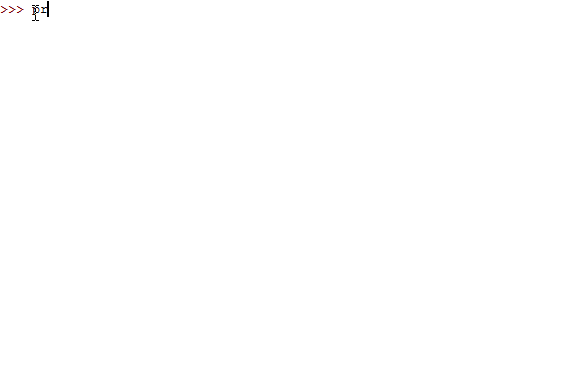2-Dimensional Lists(arrays)

Sometimes, we have to store data in a list in pairs so that each pair forms an element. For example, the forenames and surnames form such a list.

names = [["Bill","Clinton"],["Donald","Trump"],["Barack", "Obama"],["George","Bush"]]

The elements of the list should be referred to as follows:

names - Bill Clinton
names - Bill
names - George
names - Trump

#### Tuples

Tuples are sequences that cannot be updated or changed; the lists, however, can be changed. In addition, the tuples use parenthesis, whereas lists use square brackets.

Subjects = ("physics", "maths", "English", "computing")

The data in the tuple can be referred to by the index of each element: for instance, Subjects='physics' and Subjects='English'.

#### Bubble Sort

This is an algorithm that arranges a list in ascending order, by comparing each element from the beginning with its adjacent element, until the list is sorted. It's very time consuming and becomes very inefficient, if the list has been almost sorted before being subjected to bubble sort.

E.g.

Suppose, you are supposed to sort the following list in ascending order:

6, 5, 4, 3, 2, 1

The code for the bubble sort is as follows:

import time # import Python time module to use its sleep() function
def bubble_sort(the_list):
for i in range(len(the_list)):
for j in range(i+1, len(the_list)):
if the_list[j] < the_list[i]:
the_list[j], the_list[i] = the_list[i], the_list[j] #swapping the two elements
print (the_list)
time.sleep(1) #suspend the execution for 1 second to show the progress of the sequence
the_list=[6,5,4,3,2,1]
bubble_sort(the_list)

You can see bubble sort at work here:#### Euclid's Algorithm

Euclid's Algorithm is one of the oldest algorithms known to  mankind. It was found in Euclid's Elements, something dates back to 300 B.C.

When Euclid came up with the algorithm, mathematics was not known as it is today; in fact, he used geometrical methods in his discovery, as algebra was not known at that time, as a branch of mathematics.

Euclid's algorithm is used to find the HCF - Highest Common Factor - or GCD - Greatest Common Divider - of two numbers.

The corresponding flow chart for the Euclid algorithm is as follows: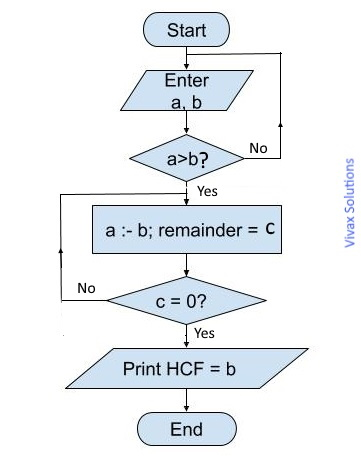The following programme shows how it determines the HCF of two numbers; you can practise it interactively.

### Writing to a text file and reading from a text file

You can easily write contents to a text file with Python and read from it, with the aid of a few commands.

Writing to a File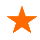#Open the file object, f, for writing.
f=open('C:\\Users\\computer name\\Documents\\my-text-file.txt','w')#Computer name is the name of the computer that you use.
#Write the contents.
f.write('Twinkle twinkle little star.\n')
f.write('How I wonder what you are.\n')
#Close the file.
f.close()

The above code write the two verses of the famous nursery rhyme to my-text-file.txt file. The open method takes two arguments: the file name, along with location and the mode, w, to show write mode. In this case, the file is written in Documents folder. After running the command, you will see the file inside the Documents folder on a Windows computer. Please make sure you close the file after writing the contents.

Appending contents to a File

If you want to add contents to the existing file, it is done as follows; the mode now changes from, w, to a.

#Open the file object, f, for appending.
f=open('C:\\Users\\computer name\\Documents\\my-text-file.txt','a')#Computer name is the name of the computer that you use.
#Write new the contents.
f.write('Up above the world so high.\n')
f.write('Like a diamond in the sky.')
#Close the file.
f.close()

Now, if you check the file in the Documents folder, you will see the entire contents.

There are a few ways to read contents from a file, with their own advantages and disadvantages. I am discussing here one way that can easily adopt in any specific requirement.

f=open('C:\\Users\\Computer Name\\Documents\\my-text-file.txt','r')
#Read the contents line by line and then print without a line break, but without blank lines.
for line in f:
print(line,end=' ')
#Close the file.
f.close()

### Searching Algorithms

An algorithm is a set of instructions to carry out a task. For example, in order to operate a microwave oven, the steps in an algorithm take up the following form:

1. Open the door.
2. Place the food on the turn-table.
3. Cover the food by a lid.
4. Close the door.
5. Set the correct heating mode.
6. Set the timer.
7. Press the start button.

We are going to study two major searching algorithm here. They are:
1) Linear Search or exhaustive search
2) Binary Search

#### Linear Search

With the linear search, we check whether a particular item, known as the key, is in an array. The key in question, in the best case scenario, may be at the very beginning of the array. In the worst case scenario, it could be at the very end of the array or not in it at all.
Array = [3, 5, 6, 9, 15]; key = 3 - the best case scenario
Array = [3, 5, 6, 9, 15]; key = 15 - the worst case scenario
Array = [3, 5, 6, 9, 15]; key = 99 - the worst case scenario
Let's experiment with a simple array to see how the linear search works, when being subjected to these two extreme cases.

E.g.

In the following example, a list of planets is used to see the process of a linear search. You can change the key and see how it works.

You can see the disadvantage of the linear search easily: if the list contains lots of items, it is going to be a tedious process to scan the whole list. If the key turns out not be in the list, it would be a sheer waste of time - and money. If a list contains 1 million items, we will need on average 500,000 steps to find out a key if it exits in the list, of course. If the list is small, however, the linear search brings results faster.

#### Binary Search

With the binary search, we check whether a particular item, known as the key, is in a sorted array. The key in question, in the best case scenario, may be at the half way point of the array. In the worst case scenario, it could not be found in the array at all.
Array = [3, 5, 6, 9, 15, 16, 17, 19, 23]; key = 15 - the best case scenario
Array = [3, 5, 6, 9, 15, 16, 17, 19, 23]; key = 99 - the worst case scenario
E.g.
To start, we first check whether the key is in the middle of the list. If not, depending on whether the key is less or greater than the middle number, we can ignore one half of the list from further searching. Let's experiment with a simple array to see how the binary search works:
Array = [3, 5, 6, 9, 15, 16, 17, 19, 23]; key = 6
Array during the first step = [3, 5, 6, 9, 15, 16, 17, 19, 23] - key is less than 15.
Array after the first step = [3, 5, 6, 9] - key is in the first half.
Array after the second step = [6, 9] - key is in the first half.
Array after the third step =  - found the key
Key is found after four steps.

E.g.

In the following example, a list of numbers is used to see the process of a binary search. You can change the key and see how it works.

You can see the advantage of a binary search over that of linear; it is far quicker. If the list has 1 million items, a key can be located after just 20 steps!

### Object Oriented Programming in Python

There are hundreds of tutorials on the subject of the OOP - Object Oriented Programming; so are the numerous challenges that pose to many of the novices.Since they all begin with a strong emphasis on the technically-heavy features of the OOP, the approach may do more harm than good for a beginner, even if the motive of the author of the tutorials in question is to present it as the best as he or she can. In this context, this tutorial is going to follow a different path, way away from the well text-muted-beaten path.That means, the features of the OOP will be discussed along with the development of the classes.

Let's consider the following situation, involving Python Turtle, the graphics creating module:

A Python Turtle programme is designed to draw polygons: it needs the name of the polygon, ranging from triangle to decagon, the number of sides and the colour for drawing. In addition, it can print the size of the external angle, internal angles and the name of the polygon, when a user needs them.

This is a real life situation. The process of modelling the above with the use of software, leads to the concept of Object Oriented Programming - OOP.

To do that, let's recognize the nouns, their attributes and verbs.

A Python Turtle programme, Polygon_Maker is designed to Draw_Polygon polygons : it needs the name of the polygon, ranging from triangle to decagon, the number of sides and the colour for drawing. In addition, it can print the size of an internal angle, external angle and the name, when a user needs them.

In OOP terminology, the interpretation of what have been highlighted is as follows:

The nouns are objects - Polygon_Maker.

The verbs are methods or functions - Draw_Polygon, Print_Int_Angle, Print_Ext_Angle, Print_Name

The attributes are properties - colour, no of sides, name

The main elements are the objects. The methods and attributes are just parts of them. In order to model real-life objects - in this case, polygons - in OOP, we create a blueprint, which is known as a class.

In the following animation, the cookie press represents the class. It makes, of course, cookies - the objects.Now, let's focus on the objects - polygons. The blueprint for the polygon objects is the Polygon_Makerclass. Its attributes and methods can be shown as follows:

Polygon_Maker

________________________________

Name

NumberofSides

Colour

________________________________

Draw()

Print_Int_Angle()

Print_Ext_Angle()

Print_Name()

Now, let's create the class and assign methods and properties to it in Python:

• We can create polygons from this class such as triangles, squares etc. This process is knows in OOP as instantiation - making instances of objects.
• Note, __init__(self) method; it is the first method to be initialized when an object is created. It's called a constructor.
• self parameter is for referring to the class, when there is a need for it.
• There are four methods in the class: Draw_Polygon(); Print_Name(); Print_Int_Angle(); Print_Ext_Angle()
• You can create any amount of polygons.

Now it's time to really practise Object Orient Programming interactively here; congratulations! you made it!!

Assuming that you understood the Object Oriented Programming well text-muted, now it's time to explore the four pillars in detail - and in a meaningful way - that the OOP is built upon. They were chosen to be at the end of the tutorial on purpose, without frightening a novice.

Abstraction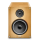The process of modelling an Polygon_Maker in programming as a class, while assigning fields, properties and methods to it, is called Abstraction in the OOP.

Thanks to abstraction, when objects are created from a class, the users will not know how objects work, as the internal working of objects remains opaque.

E.g. When you listen to a song on your stereo system, you don't know what's going on inside the speaker; you just get its functionality. This is abstraction. It's the same when you use your ignition key to start the car; most of us do not know what is going on inside the engine. In short, abstraction conceals the inside details and working from you.

Encapsulation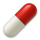The information of parameters in the methods is not visible to those who create objects from the class. This is called, encapsulation in OOP - confining data inside a capsule. It's almost like the abstraction with very little distinction. They, however, are different.

Polymorphism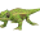This is what a chameleon is good at: changing its form from time to time, depending on the input it gets from the environment: enemy or love rivalry, luring its prey etc. Polymorphism in OOP means exactly the same. For instance, a function can behave in different ways, depending on the parameters it gets. The following example shows just that:

As you can see, Draw_Polygon() function behaves in different ways despite having the same name, depending on the parameter - or lack of it. This is polymorphism in OOP.

Inheritance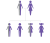This is the process of a child class inheriting a parent class. The following example shows how it is done: the Rectangle_Maker is the child class and the Polygon_Maker is the parent class. You will see the child class inheriting __init__() method of the parent class.

You can see that the child class inherits no of sides, colour and name from the parent class.

Now that you have read this tutorial, you will find the following tutorials very helpful too:

Amazon Best SellerEverything is evolving; so is the layout of a text book. By uniquely presenting the rich contents of the book, the author has elevated positive user experience of reading a text book to a new level: the examples are easy to follow and rich in standard. Highly recommended for those who want to master JavaScript and JQuery.

### Progressive Web Apps(PWA)The significance of app stores is over; progressive web apps is the next big thing. They are just websites that makes the need of going through app stores and need of storing redundant. They work offline too. If you have a reasonable understanding of HTML, CSS and JavaScript, this is the book for you to learn in no time.

### HTML5 Canvas AnimationsDavid Geary, in this book, shows how to combine JavaScript and HTML5 Canvas to produce amazing animations; he has set aside a whole chapter to teach you the role of physics in animations. If you want an in-depth understanding about HTML5 Canvas animations, this is a must read.